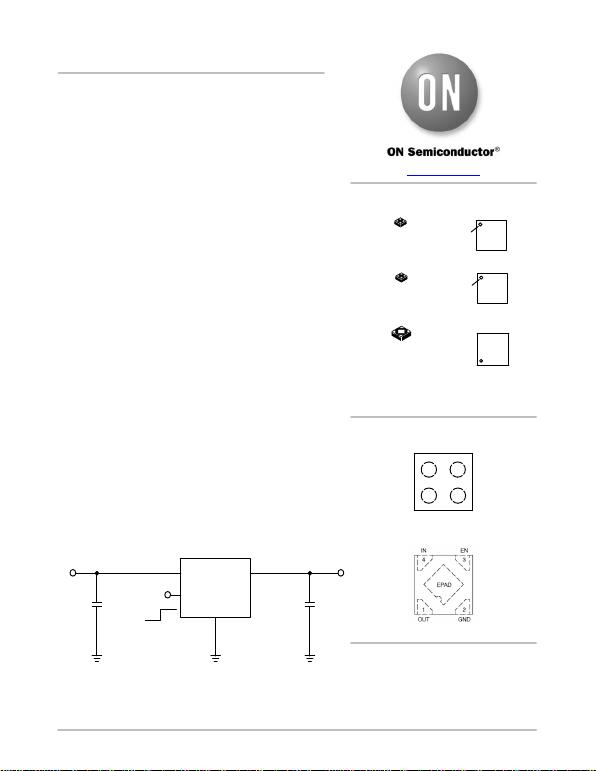© Semiconductor Components Industries, LLC, 2017
August, 2017 − Rev. 15 1Publication Order Number:
NCP160/D
NCP160
250 mA, Ultra-Low Noise
and High PSRR LDO
Regulator for RF and
Analog Circuits
The NCP160 is a linear regulator capable of supplying 250 mA
output current. Designed to meet the requirements of RF and analog
circuits, the NCP160 device provides low noise, high PSRR, low
quiescent current, and very good load/line transients. The device is
designed to work with a 1 mF input and a 1 mF output ceramic
capacitor. It is available in two thickness ultra−small 0.35P, 0.65 mm x
0.65 mm Chip Scale Package (CSP) and XDFN−4 0.65P, 1 mm x
1 mm.
Features
Operating Input Voltage Range: 1.9 V to 5.5 V
Available in Fixed Voltage Option: 1.8 V to 5.14 V
±2% Accuracy Over Load/Temperature
Ultra Low Quiescent Current Typ. 18 mA
Standby Current: Typ. 0.1 mA
Very Low Dropout: 80 mV at 250 mA
Ultra High PSRR: Typ. 98 dB at 20 mA, f = 1 kHz
Ultra Low Noise: 10 mVRMS
Stable with a 1 mF Small Case Size Ceramic Capacitors
Available in −WLCSP4 0.65 mm x 0.65 mm x 0.4 mm
−WLCSP4 0.65 mm x 0.65 mm x 0.33 mm
−XDFN4 1 mm x 1 mm x 0.4 mm
These Devices are Pb−Free, Halogen Free/BFR Free and are RoHS
Compliant
Typical Applications
Battery−powered Equipment
Wireless LAN Devices
Smartphones, Tablets
Cameras, DVRs, STB and Camcorders
IN
EN
GND
OUT
OFF
ON
Figure 1. Typical Application Schematics
VOUT
COUT
1 mF
Ceramic
VIN
NCP160
CIN
1 mF
Ceramic
WLCSP4
CASE 567KA
MARKING
DIAGRAMS
www.onsemi.com
X or XX = Specific Device Code
M = Date Code
See detailed ordering, marking and shipping information on
page 16 of this data sheet.
ORDERING INFORMATION
PIN CONNECTIONS
XDFN4
CASE 711AJ
A1 A2
B1 B2
IN OUT
EN GND
(Top View)
(Top View)
WLCSP4
CASE 567JZ
A1 X
1XX M
1
A1 X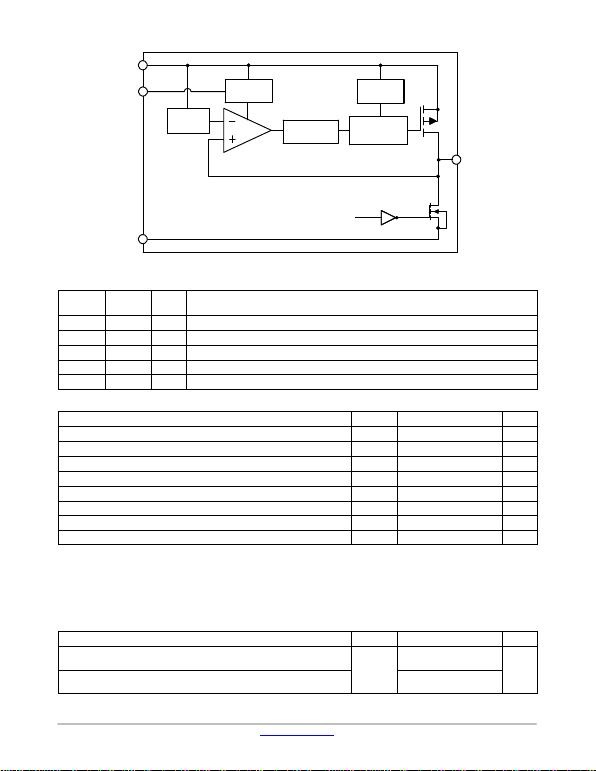NCP160
www.onsemi.com
2
Figure 2. Simplified Schematic Block Diagram
IN
THERMAL
SHUTDOWN
MOSFET
DRIVER WITH
CURRENT LIMIT
INTEGRATED
SOFT−START
BANDGAP
REFERENCE
ENABLE
LOGIC
EN
OUT
GND
EN
* ACTIVE DISCHARGE
Version A only
PIN FUNCTION DESCRIPTION
Pin No.
CSP4 Pin No.
XDFN4 Pin
Name Description
A1 4 IN Input voltage supply pin
A2 1 OUT Regulated output voltage. The output should be bypassed with small 1 mF ceramic capacitor.
B1 3 EN Chip enable: Applying VEN < 0.4 V disables the regulator, Pulling VEN > 1.2 V enables the LDO.
B2 2 GND Common ground connection
EPAD EPAD Expose pad can be tied to ground plane for better power dissipation
ABSOLUTE MAXIMUM RATINGS
Rating Symbol Value Unit
Input Voltage (Note 1) VIN −0.3 V to 6V
Output Voltage VOUT −0.3 to VIN + 0.3, max. 6 V V
Chip Enable Input VCE −0.3 to VIN + 0.3, max. 6 V V
Output Short Circuit Duration tSC unlimited s
Maximum Junction Temperature TJ150 °C
Storage Temperature TSTG −55 to 150 °C
ESD Capability, Human Body Model (Note 2) ESDHBM 2000 V
ESD Capability, Machine Model (Note 2) ESDMM 200 V
Stresses exceeding those listed in the Maximum Ratings table may damage the device. If any of these limits are exceeded, device functionality
should not be assumed, damage may occur and reliability may be af fected.
1. Refer to ELECTRICAL CHARACTERISTIS and APPLICATION INFORMATION for Safe Operating Area.
2. This device series incorporates ESD protection and is tested by the following methods:
ESD Human Body Model tested per EIA/JESD22−A114
ESD Machine Model tested per EIA/JESD22−A115
Latchup Current Maximum Rating tested per JEDEC standard: JESD78.
THERMAL CHARACTERISTICS
Rating Symbol Value Unit
Thermal Characteristics, CSP4 (Note 3)
Thermal Resistance, Junction−to−Air RqJA
108
°C/W
Thermal Characteristics, XDFN4 (Note 3)
Thermal Resistance, Junction−to−Air 198.1
3. Measured according to JEDEC board specification. Detailed description of the board can be found in JESD51−7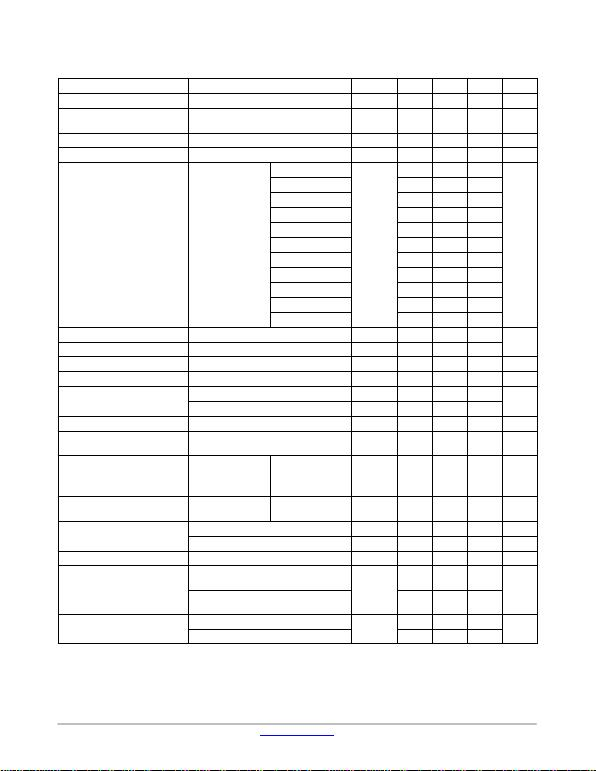NCP160
www.onsemi.com
3
ELECTRICAL CHARACTERISTICS −40°C TJ 125°C; VIN = VOUT(NOM) + 1 V; IOUT = 1 mA, CIN = COUT = 1 mF, unless otherwise
noted. VEN = 1.2 V. Typical values are at TJ = +25°C (Note 4).
Parameter Test Conditions Symbol Min Typ Max Unit
Operating Input Voltage VIN 1.9 5.5 V
Output Voltage Accuracy VIN = VOUT(NOM) + 1 V
0 mA IOUT 250 mA VOUT −2 +2 %
Line Regulation VOUT(NOM) + 1 V VIN 5.5 V LineReg 0.02 %/V
Load Regulation IOUT = 1 mA to 250 mA LoadReg 0.001 %/mA
Dropout Voltage (Note 5) IOUT = 250 mA
VOUT(NOM) = 1.8 V
VDO
180 250
mV
VOUT(NOM) = 2.5 V 110 175
VOUT(NOM) = 2.8 V 95 160
VOUT(NOM) = 2.85 V 95 160
VOUT(NOM) = 3.0 V 90 155
VOUT(NOM) = 3.2 V 85 149
VOUT(NOM) = 3.3 V 80 145
VOUT(NOM) = 3.5 V 75 140
VOUT(NOM) = 4.5 V 65 120
VOUT(NOM) = 5.0 V 75 105
VOUT(NOM) = 5.14 V 60 105
Output Current Limit VOUT = 90% VOUT(NOM) ICL 250 700 mA
Short Circuit Current VOUT = 0 V ISC 690
Quiescent Current IOUT = 0 mA IQ18 23 mA
Shutdown Current VEN 0.4 V, VIN = 4.8 V IDIS 0.01 1 mA
EN Pin Threshold Voltage EN Input Voltage “H” VENH 1.2 V
EN Input Voltage “L” VENL 0.4
EN Pull Down Current VEN = 4.8 V IEN 0.2 0.5 mA
T urn−On Time COUT = 1 mF, From assertion of VEN to
VOUT = 95% VOUT(NOM) 120 ms
Power Supply Rejection Ratio IOUT = 20 mA f = 100 Hz
f = 1 kHz
f = 10 kHz
f = 100 kHz PSRR 91
98
82
48 dB
Output Voltage Noise f = 10 Hz to 100 kHz IOUT = 1 mA
IOUT = 250 mA VN14
10 mVRMS
Thermal Shutdown Threshold Temperature rising TSDH 160 °C
Temperature falling TSDL 140 °C
Active Output Discharge Resistance VEN < 0.4 V, Version A only RDIS 280 W
Line Transient (Note 6) VIN = (VOUT(NOM) + 1 V) to (VOUT(NOM) +
1.6 V) in 30 ms, IOUT = 1 mA TranLINE
−1 mV
VIN = (VOUT(NOM) + 1.6 V) to (VOUT(NOM) +
1 V) in 30 ms, IOUT = 1 mA +1
Load Transient (Note 6) IOUT = 1 mA to 200 mA in 10 msTranLOAD −40 mV
IOUT = 200 mA to 1mA in 10 ms+40
Product parametric performance is indicated in the Electrical Characteristics for the listed test conditions, unless otherwise noted. Product
performance may not be indicated by the Electrical Characteristics if operated under different conditions.
4. Performance g u a r anteed over the indicated operating temperature range by design and/or characterization. Production tested at TA = 25°C.
Low duty cycle pulse techniques are used during the testing to maintain the junction temperature as close to ambient as possible.
5. Dropout voltage is characterized when VOUT falls 100 mV below VOUT(NOM).
6. Guaranteed by design.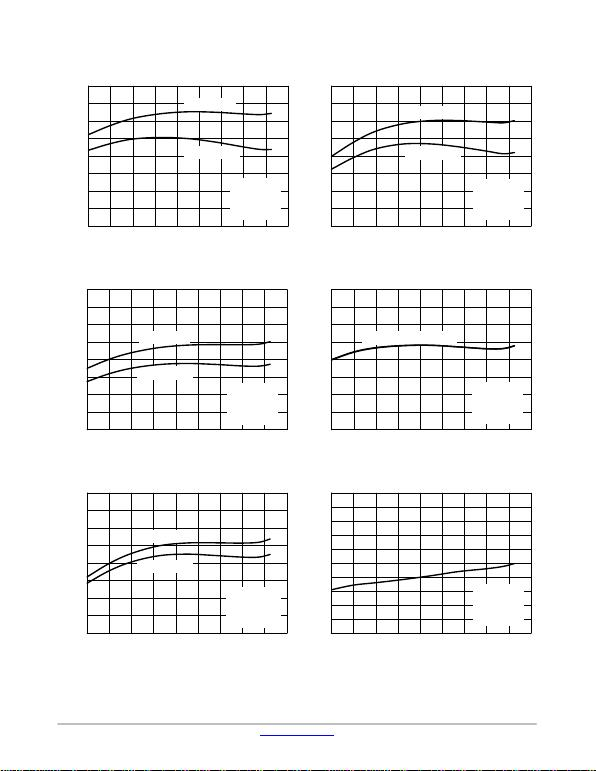NCP160
www.onsemi.com
4
TYPICAL CHARACTERISTICS
Figure 3. Output Voltage vs. Temperature −
VOUT = 1.8 V − XDFN Package Figure 4. Output Voltage vs. Temperature −
VOUT = 2.5 V − XDFN Package
TJ, JUNCTION TEMPERATURE (°C) TJ, JUNCTION TEMPERATURE (°C)
1201008060200−20−40
1.780
1.785
1.790
1.810
1.800
1.805
1.815
1.820
1201008060400−20−40
2.480
2.485
2.490
2.495
2.500
2.510
2.515
2.520
Figure 5. Output Voltage vs. Temperature −
VOUT = 3.3 V − XDFN Package Figure 6. Output Voltage vs. Temperature −
VOUT = 3.3 V − CSP Package
TJ, JUNCTION TEMPERATURE (°C) TJ, JUNCTION TEMPERATURE (°C)
1201008040200−20−40
3.25
3.26
3.27
3.28
3.29
3.31
3.32
3.33
1201008060400−20−40
3.27
3.28
3.29
3.30
3.31
3.33
3.34
3.35
Figure 7. Output Voltage vs. Temperature −
VOUT = 5.14 V − XDFN Package Figure 8. Line Regulation vs. Temperature −
VOUT = 1.8 V
TJ, JUNCTION TEMPERATURE (°C) TJ, JUNCTION TEMPERATURE (°C)
1201006040200−20−40
5.11
5.12
5.13
5.14
5.15
5.17
5.18
5.19
1201008060200−20−40
0
0.001
0.003
0.004
0.005
0.007
0.009
0.010
VOUT, OUTPUT VOLTAGE (V)
VOUT, OUTPUT VOLTAGE (V)
VOUT, OUTPUT VOLTAGE (V)
VOUT, OUTPUT VOLTAGE (V)
VOUT, OUTPUT VOLTAGE (V)
REGLINE, LINE REGULATION (%/V)
40 140
1.795
IOUT = 10 mA
IOUT = 250 mA
VIN = 2.8 V
VOUT = 1.8 V
CIN = 1 mF
COUT = 1 mF
20 140
2.505
IOUT = 10 mA
IOUT = 250 mA
VIN = 3.5 V
VOUT = 2.5 V
CIN = 1 mF
COUT = 1 mF
IOUT = 10 mA
IOUT = 250 mA
VIN = 4.3 V
VOUT = 3.3 V
CIN = 1 mF
COUT = 1 mF
3.30
60 140 20 140
VIN = 4.3 V
VOUT = 3.3 V
CIN = 1 mF
COUT = 1 mF
IOUT = 10 mA and 250 mA
3.32
80 140
IOUT = 10 mA
IOUT = 250 mA
VIN = 5.5 V
VOUT = 5.14 V
CIN = 1 mF
COUT = 1 mF
5.16
40 140
0.002
0.006
0.008
VIN = 2.8 V
VOUT = 1.8 V
CIN = 1 mF
COUT = 1 mF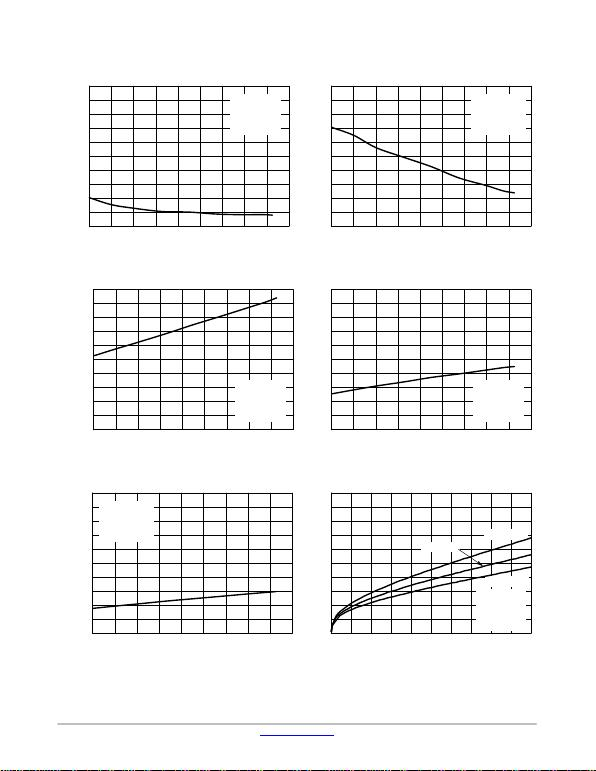NCP160
www.onsemi.com
5
TYPICAL CHARACTERISTICS
Figure 9. Line Regulation vs. Temperature −
VOUT = 3.3 V Figure 10. Line Regulation vs. Temperature −
VOUT = 5.14 V
TJ, JUNCTION TEMPERATURE (°C) TJ, JUNCTION TEMPERATURE (°C)
1201008060200−20−40
0
0.001
0.003
0.004
0.006
0.007
0.009
0.010
1201008060200−20−40
0
0.002
0.004
0.006
0.012
0.014
0.016
0.020
Figure 11. Load Regulation vs. Temperature −
VOUT = 1.8 V Figure 12. Load Regulation vs. Temperature −
VOUT = 3.3 V
TJ, JUNCTION TEMPERATURE (°C) TJ, JUNCTION TEMPERATURE (°C)
120806040200−20−40
0
0.0002
0.0006
0.0008
0.0010
0.0014
0.0016
0.0020
1201008060200−20−40
0
0.0002
0.0006
0.0008
0.0010
0.0014
0.0016
0.0020
Figure 13. Load Regulation vs. Temperature −
VOUT = 5.14 V Figure 14. Ground Current vs. Load Current −
VOUT = 1.8 V
TJ, JUNCTION TEMPERATURE (°C) IOUT, OUTPUT CURRENT (mA)
1201008040200−20−40
0
0.0002
0.0006
0.0008
0.0012
0.0014
0.0018
0.0020
22517515012510075250
0
0.15
0.45
0.60
0.90
1.05
1.35
1.50
REGLINE, LINE REGULATION (%/V)REGLOAD, LOAD REGULATION (%/mA)
IGND, GROUND CURRENT (mA)
40 140
0.002
0.005
0.008
VIN = 4.3 V
VOUT = 3.3 V
CIN = 1 mF
COUT = 1 mF
40 140
0.008
0.010
0.018 VIN = 5.5 V
VOUT = 5.14 V
CIN = 1 mF
COUT = 1 mF
100 140
0.0004
0.0012
0.0018
VIN = 2.8 V
VOUT = 1.8 V
CIN = 1 mF
COUT = 1 mF
40 140
0.0004
0.0012
0.0018
VIN = 4.3 V
VOUT = 3.3 V
CIN = 1 mF
COUT = 1 mF
60 140
0.0004
0.0010
0.0016
VIN = 5.5 V
VOUT = 5.14 V
CIN = 1 mF
COUT = 1 mF
0.30
0.75
1.20
50 200 250
VIN = 2.8 V
VOUT = 1.8 V
CIN = 1 mF
COUT = 1 mF
TJ = 125°C
TJ = 25°C
TJ = −40°C
REGLINE, LINE REGULATION (%/V)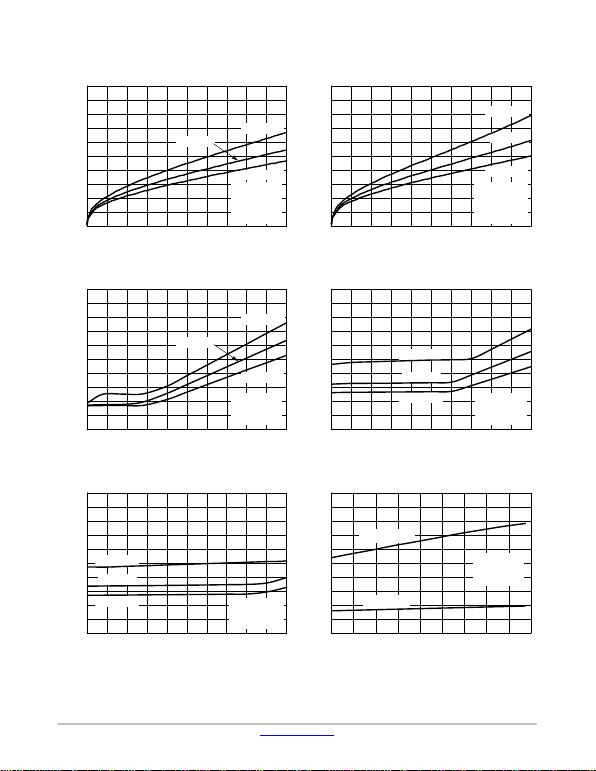NCP160
www.onsemi.com
6
TYPICAL CHARACTERISTICS
Figure 15. Ground Current vs. Load Current −
VOUT = 3.3 V Figure 16. Ground Current vs. Load Current −
VOUT = 5.14 V
IOUT, OUTPUT CURRENT (mA) IOUT, OUTPUT CURRENT (mA)
2251751501257550250
0
0.15
0.45
0.60
0.90
1.05
1.35
1.50
22520015012510050250
0
0.15
0.45
0.60
0.90
1.05
1.35
1.50
Figure 17. Dropout Voltage vs. Load Current −
VOUT = 1.8 V Figure 18. Dropout Voltage vs. Load Current −
VOUT = 3.3 V
IOUT, OUTPUT CURRENT (mA) IOUT, OUTPUT CURRENT (mA)
2252001501007550250
0
25
75
100
150
175
225
250
22520015012510050250
0
15
45
60
75
120
150
Figure 19. Dropout Voltage vs. Load Current −
VOUT = 5.14 V Figure 20. Dropout Voltage vs. Temperature−
VOUT = 1.8 V
IOUT, OUTPUT CURRENT (mA) TJ, JUNCTION TEMPERATURE (°C)
22520015012510050250
0
15
45
60
90
105
135
150
1201008060200−20−40
0
25
75
100
150
175
225
250
IGND, GROUND CURRENT (mA)
IGND, GROUND CURRENT (mA)
VDROP, DROPOUT VOLTAGE (mV)
VDROP, DROPOUT VOLTAGE (mV)
VDROP, DROPOUT VOLTAGE (mV)
VDROP, DROPOUT VOLTAGE (mV)
VIN = 4.3 V
VOUT = 3.3 V
CIN = 1 mF
COUT = 1 mF
TJ = 125°C
TJ = 25°C
TJ = −40°C
100 200 250
0.30
0.75
1.20
75 175 250
0.30
0.75
1.20
VIN = 5.5 V
VOUT = 5.14 V
CIN = 1 mF
COUT = 1 mF
TJ = 125°C
TJ = 25°C
TJ = −40°C
VOUT = 1.8 V
CIN = 1 mF
COUT = 1 mF
TJ = 125°C
TJ = 25°C
TJ = −40°C
50
125
200
125 175 250
VOUT = 3.3 V
CIN = 1 mF
COUT = 1 mF
TJ = 125°C
TJ = 25°C
TJ = −40°C
75 175 250
30
105
135
90
75 175 250
30
75
120
VOUT = 5.14 V
CIN = 1 mF
COUT = 1 mF
TJ = 125°C
TJ = 25°C
TJ = −40°C
40 140
50
125
200
IOUT = 0 mA
IOUT = 250 mA
VOUT = 1.8 V
CIN = 1 mF
COUT = 1 mF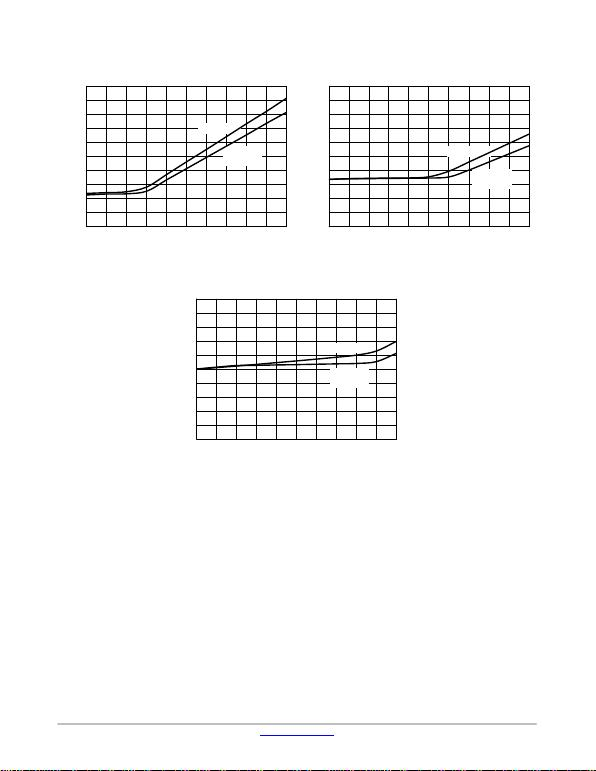NCP160
www.onsemi.com
7
TYPICAL CHARACTERISTICS
Figure 21. Comparison Dropout for XDFN and
CSP – 1.8 V
IOUT, OUTPUT CURRENT (mA)
2252001501251005025
200
V
DROP
, DROPOUT VOLTAGE (mV)
75 175 250
XDFN
CSP4
Package
180
160
140
120
100
80
60
40
20
00
Figure 22. Comparison Dropout for XDFN and
CSP – 3.3 V
IOUT, OUTPUT CURRENT (mA)
2252001501251005025
150
VDROP, DROPOUT VOLTAGE (mV)
75 175 25
0
0
XDFN
CSP4
Package
135
120
105
90
75
60
45
30
15
0
Figure 23. Comparison Dropout for XDFN and
CSP – 5.14 V
IOUT, OUTPUT CURRENT (mA)
2252001501251005025
VDROP, DROPOUT VOLTAGE (mV)
75 175 250
100
80
60
40
20
00
XDFN
CSP4
Package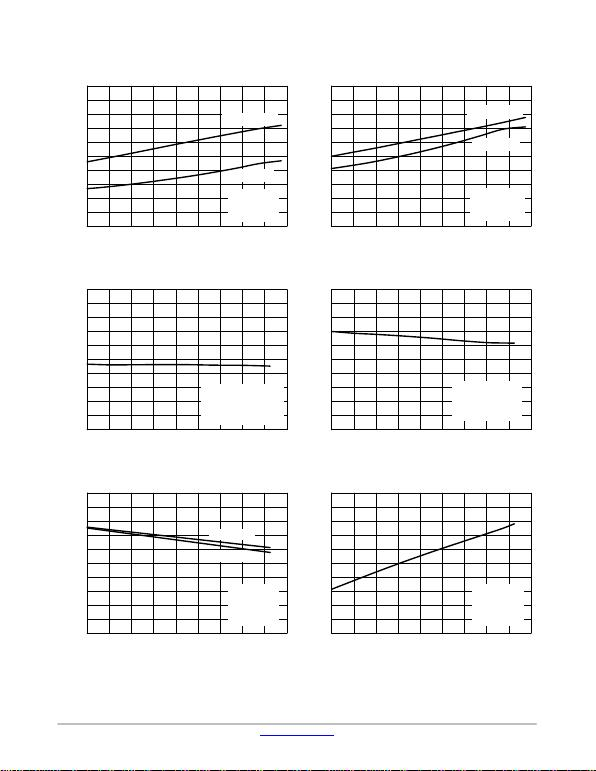NCP160
www.onsemi.com
8
TYPICAL CHARACTERISTICS
Figure 24. Dropout Voltage vs. Temperature−
VOUT = 3.3 V Figure 25. Dropout Voltage vs. Temperature−
VOUT = 5.14 V
TJ, JUNCTION TEMPERATURE (°C) TJ, JUNCTION TEMPERATURE (°C)
1201008060200−20−40
0
15
45
60
90
105
135
150
1201008060400−20−40
0
10
30
40
50
70
90
100
Figure 26. Current Limit vs. Temperature Figure 27. Short Circuit Current vs.
Temperature
TJ, JUNCTION TEMPERATURE (°C) TJ, JUNCTION TEMPERATURE (°C)
1201008060400−20−40
650
670
680
690
710
720
740
750
1201008060400−20−40
600
610
630
640
660
670
690
700
Figure 28. Enable Threshold Voltage vs.
Temperature Figure 29. Enable Current Temperature
TJ, JUNCTION TEMPERATURE (°C) TJ, JUNCTION TEMPERATURE (°C)
1201008060200−20−40
0
0.1
0.3
0.4
0.6
0.7
0.9
1.0
1201008060400−20−40
0
0.05
0.10
0.20
0.30
0.35
0.40
0.50
VDROP, DROPOUT VOLTAGE (mV)
VDROP, DROPOUT VOLTAGE (mV)
ICL, CURRENT LIMIT (mA)
ICL, SHORT CIRCUIT CURRENT (mA)
VEN, ENABLE VOLTAGE THRESHOLD (V)
IEN, ENABLE PIN CURRENT (mA)
40 140
30
75
120
IOUT = 0 mA
IOUT = 250 mA
VOUT = 3.3 V
CIN = 1 mF
COUT = 1 mF
20 140
20
60
80
IOUT = 0 mA
IOUT = 250 mA
VOUT = 5.14 V
CIN = 1 mF
COUT = 1 mF
20 140
660
700
730
VIN = 4.3 V
VOUT = 90% VOUT(nom)
CIN = 1 mF
COUT = 1 mF
20 140
620
650
680
VIN = 4.3 V
VOUT = 0 V (Short)
CIN = 1 mF
COUT = 1 mF
40 140
0.2
0.5
0.8
OFF −> ON
ON −> OFF
VIN = 4.3 V
VOUT = 3.3 V
CIN = 1 mF
COUT = 1 mF
VIN = 4.3 V
VOUT = 3.3 V
CIN = 1 mF
COUT = 1 mF
20 140
0.15
0.25
0.45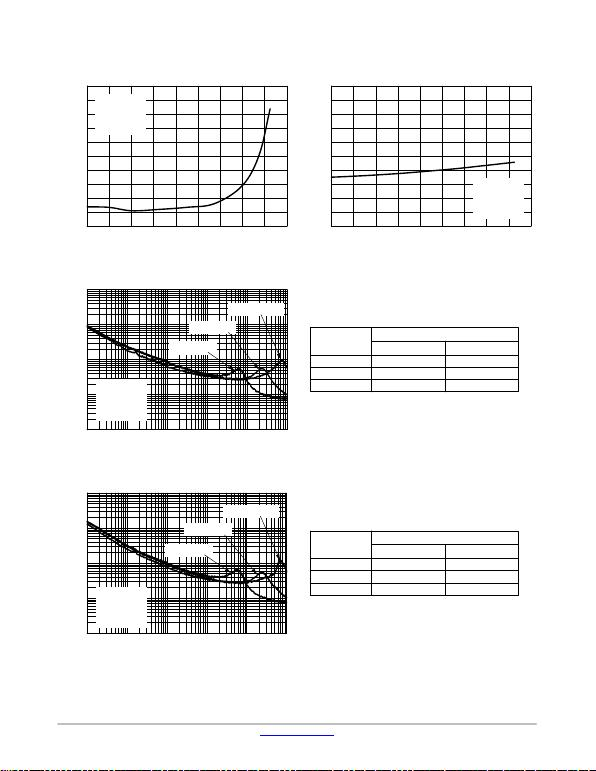NCP160
www.onsemi.com
9
TYPICAL CHARACTERISTICS
Figure 30. Disable Current vs. Temperature Figure 31. Discharge Resistivity vs.
Temperature
TJ, JUNCTION TEMPERATURE (°C) TJ, JUNCTION TEMPERATURE (°C)
1201008060200−20−40
0
10
30
40
60
70
90
100
1201008060400−20−40
200
220
230
240
260
270
290
300
Figure 32. Output Voltage Noise Spectral Density − VOUT = 1.8 V
FREQUENCY (kHz)
10001001010.10.01
1
10
100
1000
10,000
Figure 33. Output Voltage Noise Spectral Density − VOUT = 3.3 V
FREQUENCY (kHz)
10001001010.10.01
1
10
100
1000
10,000
IDIS, DISABLE CURRENT (nA)
RDIS, DISCHARGE RESISTIVITY
OUTPUT VOLTAGE NOISE (nV/Hz)
20
50
80
40 140
VIN = 4.3 V
VOUT = 3.3 V
CIN = 1 mF
COUT = 1 mF
20 140
210
250
280
VIN = 4.3 V
VOUT = 3.3 V
CIN = 1 mF
COUT = 1 mF
1 mA 14.62 14.10
10 mA 11.12 10.48
250 mA 10.37 9.82
10 Hz − 100 kHz 100 Hz − 100 kHz
RMS Output Noise (mV)
IOUT
1 mA 16.9 15.79
10 mA 12.64 11.13
250 mA 11.96 10.64
10 Hz − 100 kHz 100 Hz − 100 kHz
RMS Output Noise (mV)
IOUT
OUTPUT VOLTAGE NOISE (nV/Hz)
VIN = 2.8 V
VOUT = 1.8 V
CIN = 1 mF
COUT = 1 mF
IOUT = 1 mA
IOUT = 250 mA
IOUT = 10 mA
VIN = 4.3 V
VOUT = 3.3 V
CIN = 1 mF
COUT = 1 mF
IOUT = 1 mA
IOUT = 250 mA
IOUT = 10 mA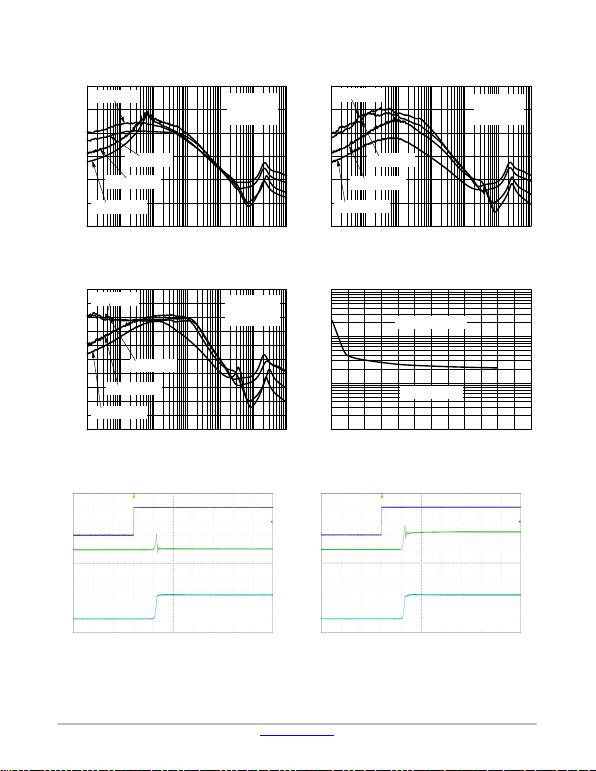NCP160
www.onsemi.com
10
TYPICAL CHARACTERISTICS
Figure 34. Power Supply Rejection Ratio,
VOUT = 1.8 V Figure 35. Power Supply Rejection Ratio,
VOUT = 3.3 V
FREQUENCY (kHz) FREQUENCY (kHz)
10k1k1001010.10.01
0
20
40
60
80
100
120
10k1k1001010.10.01
0
20
40
60
80
100
120
Figure 36. Power Supply Rejection Ratio,
VOUT = 5.14 V Figure 37. Stability vs. ESR
FREQUENCY (kHz) IOUT, OUTPUT CURRENT (mA)
10k1k1001010.10.01
0
10
30
40
50
70
80
100
300250200150100500
0.1
1
10
100
Figure 38. Enable Turn−on Response −
COUT = 1 mF, IOUT = 10 mA Figure 39. Enable Turn−on Response −
COUT = 1 mF, IOUT = 250 mA
100 ms/div 100 ms/div
RR, RIPPLE REJECTION (dB)
RR, RIPPLE REJECTION (dB)
RR, RIPPLE REJECTION (dB)
ESR (W)
500 mV/div
VIN = 2.5 V
VOUT = 1.8 V
COUT = 1 mF
IOUT = 10 mA
IOUT = 250 mA
IOUT = 20 mA
IOUT = 100 mA
VIN = 3.6 V
VOUT = 3.3 V
COUT = 1 mF
IOUT = 10 mA
IOUT = 250 mA
IOUT = 100 mA
IOUT = 20 mA
VIN = 5.5 V
VOUT = 5.14 V
COUT = 1 mF
IOUT = 10 mA
IOUT = 250 mA
IOUT = 20 mA
IOUT = 100 mA
20
60
90
Unstable Operation
Stable Operation
VIN = 2.8 V, VOUT = 1.8 V
CIN = 1 mF (MLCC)
COUT = 1 mF (MLCC)
VEN
IINPUT
VOUT
1 V/div
500 mV/div1 V/div
200 mA/div
200 mA/div
VIN = 2.8 V, VOUT = 1.8 V
CIN = 1 mF (MLCC)
COUT = 1 mF (MLCC)
VEN
IINPUT
VOUT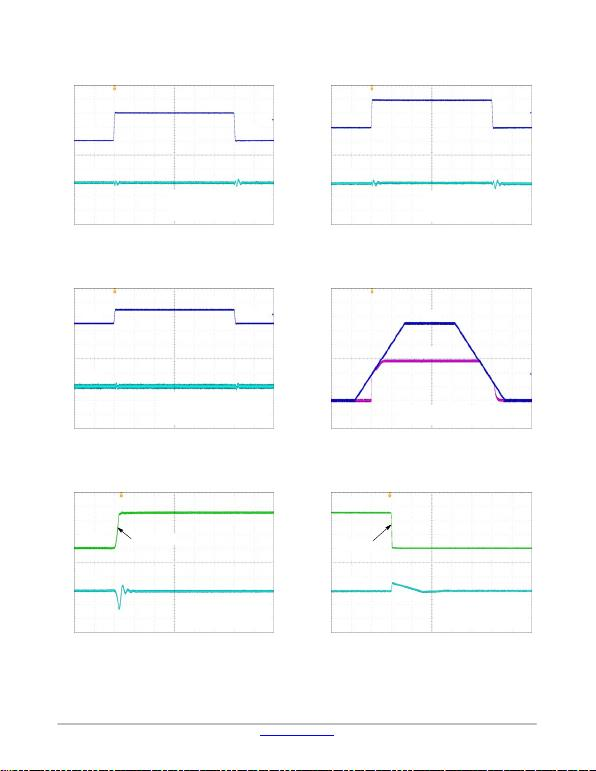NCP160
www.onsemi.com
11
TYPICAL CHARACTERISTICS
Figure 40. Line Transient Response −
VOUT = 1.8 V Figure 41. Line Transient Response −
VOUT = 3.3 V
20 ms/div 20 ms/div
Figure 42. Line Transient Response −
VOUT = 5.14 V Figure 43. Turn−on/off − Slow Rising VIN
20 ms/div 4 ms/div
Figure 44. Load Transient Response −
1 mA to 250 mA − VOUT = 1.8 V Figure 45. Load Transient Response −
250 mA to 1 mA − VOUT = 1.8 V
4 ms/div 20 ms/div
500 mV/div
VOUT = 1.8 V, IOUT = 10 mA
CIN = 1 mF (MLCC)
COUT = 1 mF (MLCC)
VIN
3.3 V
VOUT
10 mV/div
2.3 V
500 mV/div10 mV/div
VOUT = 3.3 V, IOUT = 10 mA
CIN = 1 mF (MLCC)
COUT = 1 mF (MLCC)
4.8 V
3.8 V
1 V/div100 mA/div50 mV/div
200 mV/div
VOUT = 5.14 V, IOUT = 10 mA
CIN = 1 mF (MLCC)
COUT = 1 mF (MLCC)
VIN
5.5 V
VOUT
10 mV/div
5.3 V
VOUT = 2.8 V, CIN = 1 mF (MLCC),
IOUT = 10 mA, COUT = 1 mF (MLCC)
VIN
VOUT
100 mA/div50 mV/div
VIN = 2.8 V, VOUT = 1.8 V
CIN = 1 mF (MLCC)
COUT = 1 mF (MLCC)
IOUT
VOUT
tRISE = 1 ms
VIN = 2.8 V, VOUT = 1.8 V
CIN = 1 mF (MLCC)
COUT = 1 mF (MLCC)
IOUT
VOUT
tFALL = 1 ms
VIN
VOUT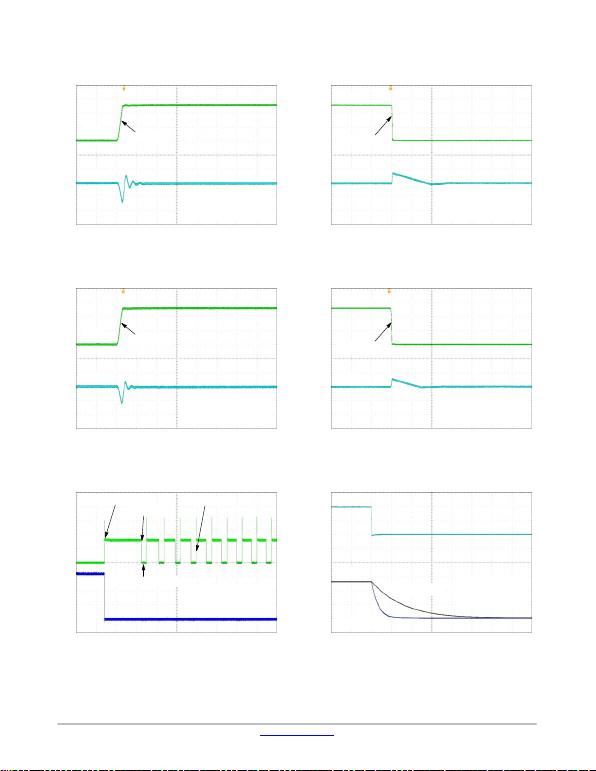NCP160
www.onsemi.com
12
TYPICAL CHARACTERISTICS
Figure 46. Load Transient Response −
1 mA to 250 mA − VOUT = 3.3 V Figure 47. Load Transient Response −
250 mA to 1 mA − VOUT = 3.3 V
4 ms/div 20 ms/div
Figure 48. Load Transient Response −
1 mA to 250 mA − VOUT = 5.14 V Figure 49. Load Transient Response −
250 mA to 1 mA − VOUT = 5.14 V
4 ms/div 20 ms/div
Figure 50. Short Circuit and Thermal
Shutdown Figure 51. Enable Turn−off
10 ms/div 400 ms/div
100 mA/div50 mV/div
VIN = 4.3 V, VOUT = 3.3 V
CIN = 1 mF (MLCC)
COUT = 1 mF (MLCC)
IOUT
VOUT
tRISE = 1 ms
100 mA/div50 mV/div
VIN = 4.3 V, VOUT = 3.3 V
CIN = 1 mF (MLCC)
COUT = 1 mF (MLCC)
IOUT
VOUT
tFALL = 1 ms
100 mA/div50 mV/div
VIN = 5.5 V, VOUT = 5.14 V
CIN = 1 mF (MLCC)
COUT = 1 mF (MLCC)
IOUT
VOUT
tFALL = 1 ms
100 mA/div50 mV/div
VIN = 5.5 V, VOUT = 5.14 V
CIN = 1 mF (MLCC)
COUT = 1 mF (MLCC)
IOUT
VOUT
tRISE = 1 ms
500 mV/div1 V/div
VIN = 3.8 V
VOUT = 2.8 V
CIN = 1 mF (MLCC)
VEN
VOUT
COUT = 1 mF
COUT = 4.7 mF
500 mA/div1 V/div
IOUT
VOUT
Short Circuit Event
Overheating
Thermal Shutdown
TSD Cycling
VIN = 5.5 V, VOUT = 3.3 V
CIN = 1 mF (MLCC)
COUT = 1 mF (MLCC)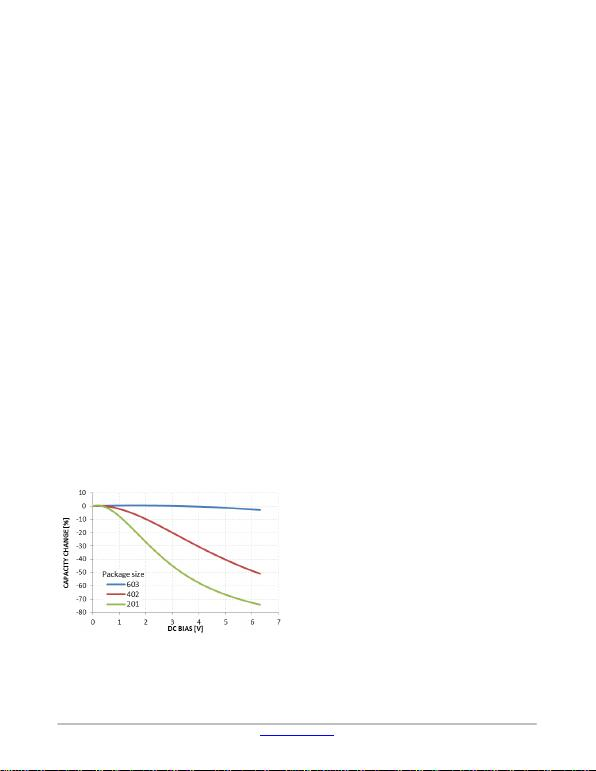NCP160
www.onsemi.com
13
APPLICATIONS INFORMATION
General
The NCP160 is an ultra−low noise 250 mA low dropout
regulator designed to meet the requirements of RF
applications and high performance analog circuits. The
NCP160 device provides very high PSRR and excellent
dynamic response. In connection with low quiescent current
this device is well suitable for battery powered application
such as cell phones, tablets and other. The NCP160 is fully
protected i n case of current overload, output short circuit and
overheating.
Input Capacitor Selection (CIN)
Input capacitor connected as close as possible is necessary
for ensure device stability. The X7R or X5R capacitor
should be used for reliable performance over temperature
range. The value of the input capacitor should be 1 mF or
greater to ensure the best dynamic performance. This
capacitor will provide a low impedance path for unwanted
AC signals or noise modulated onto constant input voltage.
There is no requirement for the ESR of the input capacitor
but it is recommended to use ceramic capacitors for their low
ESR and ESL. A good input capacitor will limit the
influence of input trace inductance and source resistance
during sudden load current changes.
Output Decoupling (COUT)
The NCP160 requires an output capacitor connected as
close as possible to the output pin of the regulator. The
recommended capacitor value is 1 mF and X7R or X5R
dielectric due to its low capacitance variations over the
specified temperature range. The NCP160 is designed to
remain stable with minimum ef fective capacitance of 0.7 mF
to account for changes with temperature, DC bias and
package size. Especially for small package size capacitors
such a s 0201 the ef fective capacitance drops rapidly with the
applied DC bias. Please refer Figure 52.
Figure 52. Capacity vs DC Bias Voltage
There is no requirement for the minimum value of
Equivalent Series Resistance (ESR) for the COUT but the
maximum value of ESR should be less than 2 W. Larger
output capacitors and lower ESR could improve the load
transient response or high frequency PSRR. It is not
recommended to use tantalum capacitors on the output due
to their large ESR. The equivalent series resistance of
tantalum capacitors is also strongly dependent on the
temperature, increasing at low temperature.
Enable Operation
The NCP160 uses the EN pin to enable/disable its device
and to deactivate/activate the active discharge function.
If the EN pin voltage is <0.4 V the device is guaranteed to
be disabled. The pass transistor is turned−off so that there is
virtually no current flow between the IN and OUT. The
active dischar ge transistor is active so that the output voltage
VOUT is pulled to GND through a 280 Ω resistor. In the
disable state the device consumes as low as typ. 10 nA from
the VIN.
If the EN pin voltage >1.2 V the device is guaranteed to
be enabled. The NCP160 regulates the output voltage and
the active discharge transistor is turned−off.
The EN pin has internal pull−down current source with
typ. value of 200 nA which assures that the device is
turned−off when the EN pin is not connected. In the case
where the EN function isn’t required the EN should be tied
directly to IN.
Output Current Limit
Output Current is internally limited within the IC to a
typical 700 mA. The NCP60 will source this amount of
current measured with a voltage drops on the 90% of the
nominal VOUT. If the Output Voltage is directly shorted to
ground (VOUT = 0 V), the short circuit protection will limit
the output current to 690 mA (typ). The current limit and
short circuit protection will work properly over whole
temperature range and also input voltage range. There is no
limitation for the short circuit duration.
Thermal Shutdown
When the die temperature exceeds the Thermal Shutdown
threshold ( T SD * 160°C typical), Thermal Shutdown event
is detected and the device is disabled. The IC will remain in
this state until the die temperature decreases below the
Thermal Shutdown Reset threshold (TSDU − 140°C typical).
Once the IC temperature falls below the 140°C the LDO is
enabled again. The thermal shutdown feature provides the
protection from a catastrophic device failure due to
accidental overheating. This protection is not intended to be
used as a substitute for proper heat sinking.
Power Dissipation
As power dissipated in the NCP160 increases, it might
become necessary to provide some thermal relief. The
maximum power dissipation supported by the device is
dependent upon board design and layout. Mounting pad
configuration on the PCB, the board material, and the
ambient temperature affect the rate of junction temperature
rise for the part.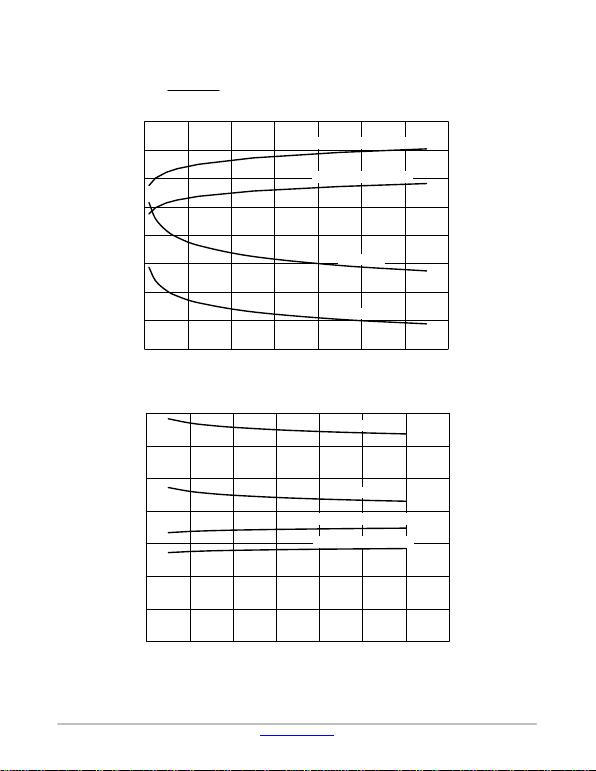NCP160
www.onsemi.com
14
The maximum power dissipation the NCP160 can handle
is given by:
PD(MAX) +ƪ125oC*TAƫ
qJA (eq. 1)
The power dissipated by the NCP160 for given
application conditions can be calculated from the following
equations:
PD[VIN @IGND )IOUTǒVIN *VOUTǓ(eq. 2)
Figure 53. qJA and PD (MAX) vs. Copper Area (CSP4)
0
0.2
0.4
0.6
0.8
1.0
1.2
1.4
1.6
80
90
100
110
120
130
140
150
160
0 100 200 300 400 500 600 700
PCB COPPER AREA (mm2)
qJA, JUNCTION TO AMBIENT THERMAL RESISTANCE (°C/W)
PD(MAX), MAXIMUM POWER DISSIPATION (W)
qJA, 2 oz Cu
qJA, 1 oz Cu
PD(MAX), TA = 25°C, 1 oz Cu
PD(MAX), TA = 25°C, 2 oz Cu
Figure 54. qJA and PD (MAX) vs. Copper Area (XDFN44)
0.3
0.4
0.5
0.6
0.8
0.7
0.9
1.0
150
160
170
180
190
200
210
220
0 100 200 300 400 500 600 700
PCB COPPER AREA (mm2)
qJA, JUNCTION TO AMBIENT THERMAL RESISTANCE (°C/W)
PD(MAX), MAXIMUM POWER DISSIPATION (W)
qJA, 2 oz Cu
qJA, 1 oz Cu
PD(MAX), TA = 25°C, 1 oz Cu
PD(MAX), TA = 25°C, 2 oz CuNCP160
www.onsemi.com
15
Reverse Current
The PMOS pass transistor has an inherent body diode
which will be forward biased in the case that VOUT > VIN.
Due to this fact in cases, where the extended reverse current
condition can be anticipated the device may require
Power Supply Rejection Ratio
The NCP160 features very high Power Supply Rejection
ratio. If desired the PSRR at higher frequencies in the range
100 kHz – 10 MHz can be tuned by the selection of COUT
capacitor and proper PCB layout.
Turn−On Time
The turn−on time is defined as the time period from EN
assertion to the point in which VOUT will reach 98% of its
nominal value. This time is dependent on various
application conditions such as VOUT(NOM), COUT, TA.
PCB Layout Recommendations
To obtain good transient performance and good regulation
characteristics place CIN and COUT capacitors close to the
device pins and make the PCB traces wide. In order to
minimize the solution size, use 0402 or 0201 capacitors with
appropriate capacity. Larger copper area connected to the
pins will also improve the device thermal resistance. The
actual power dissipation can be calculated from the equation
above (Equation 2). Expose pad can be tied to the GND pin
for improvement power dissipation and lower device
temperature.